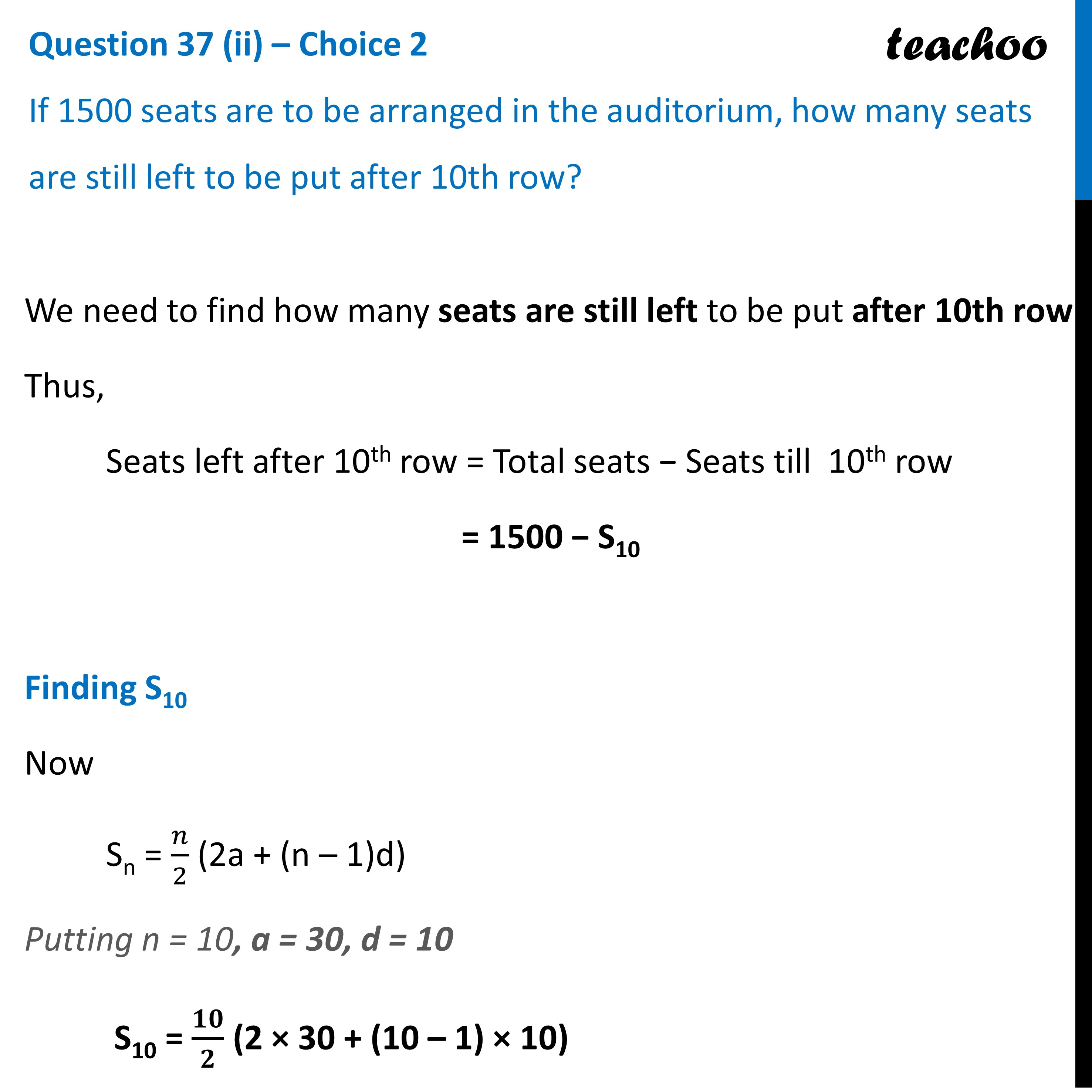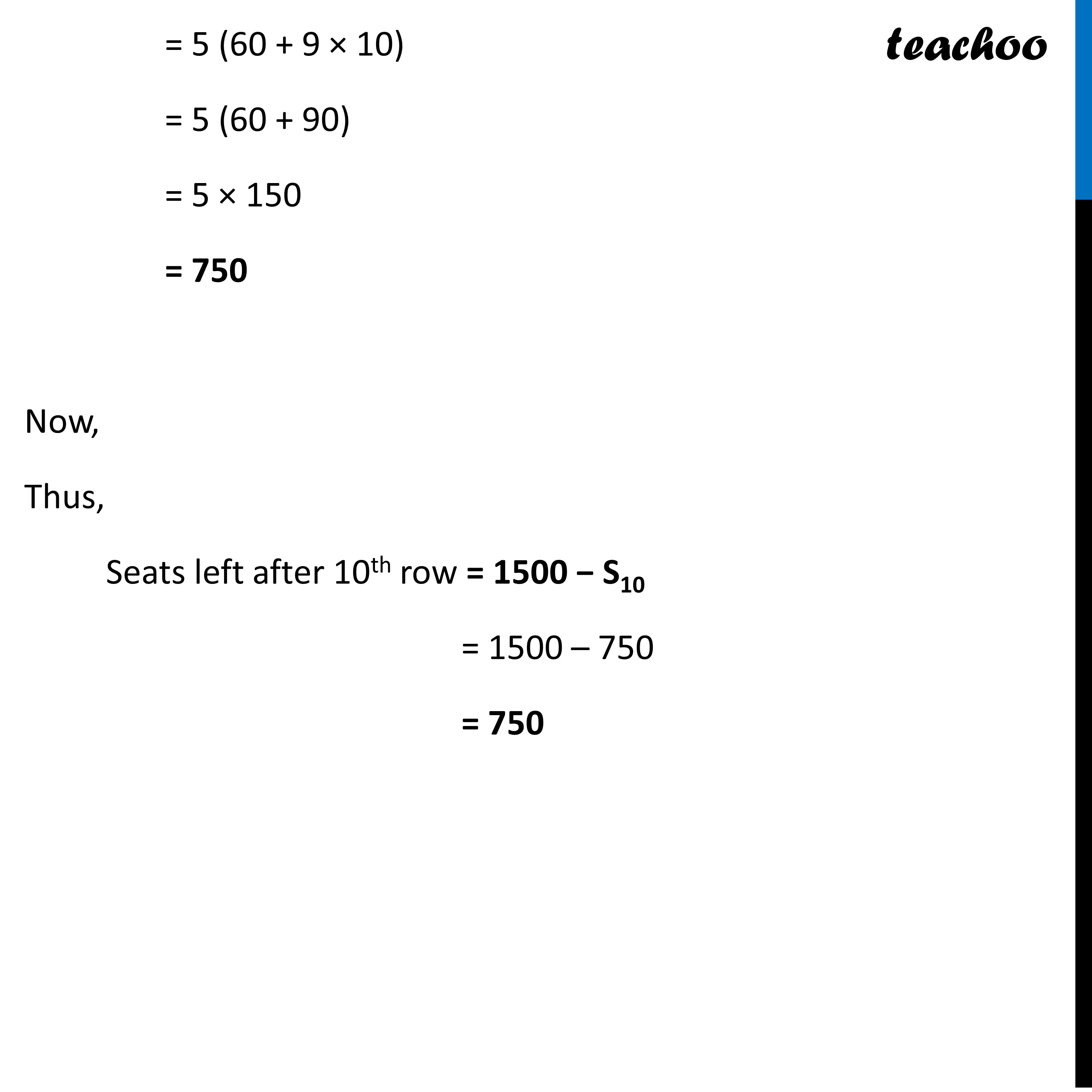CBSE Class 10 Sample Paper for 2023 Boards - Maths Standard

Class 10
Solutions of Sample Papers for Class 10 Boards

## If 1500 seats are to be arranged in the auditorium, how many seats are still left to be put after 10th row?Learn in your speed, with individual attention - Teachoo Maths 1-on-1 Class

### Transcript

Question 37 (ii) – Choice 2 If 1500 seats are to be arranged in the auditorium, how many seats are still left to be put after 10th row? We need to find how many seats are still left to be put after 10th row Thus, Seats left after 10th row = Total seats − Seats till 10th row = 1500 − S10 Finding S10 Now Sn = 𝑛/2 (2a + (n – 1)d) Putting n = 10, a = 30, d = 10 S10 = 𝟏𝟎/𝟐 (2 × 30 + (10 – 1) × 10) = 5 (60 + 9 × 10) = 5 (60 + 90) = 5 × 150 = 750 Now, Thus, Seats left after 10th row = 1500 − S10 = 1500 – 750 = 750